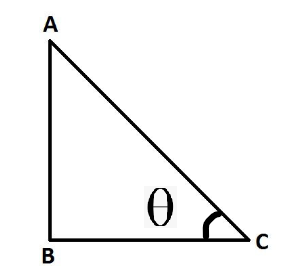Evaluate 2 tan2 45° + cos2 30° – sin2 60°

• Last Updated : 12 Oct, 2021

Trigonometry is a branch of mathematics that generally deals with triangles and angles associated with it. Trigonometry helps in understanding the properties of triangles and the real-life application of the triangle properties. Trigonometry consists of two words namely trigon and geometry, which itself tells the meaning in simple form i.e. the geometry of triangles (where trigon refers to triangles).

Trigonometry helps in understanding how to find missing angles provided the length of the sides of triangles are given, or it can also be used to find the value of certain angles like 0, 30, 45, 60, 90, etc.

Trigonometric Ratios

There are six functions or trigonometric ratios of an angle that are present in trigonometry. Their names and abbreviations are sine (sin), cosine (cos), tangent (tan), cotangent (cot), secant (sec), and cosecant (csc). One important thing to note here is that trigonometric formulas work only on the right-angle triangle. Let’s see the below image.In this right-angle triangle, side AC is known as hypotenuse. Side BC is known as the base of the triangle. Side AB is known as the height of the triangle. From the above triangle, we can list out formulas as above,

• sin∅ = AB/AC
• cos∅ = BC/AC
• tan∅ = AB/BC
• cot∅ = BC/AC
• cosec∅ = AC/BC
• sec∅ = BC/AC

Basic formulas of trigonometric ratios

• Tan ∅ = sin ∅/cos ∅
• sin ∅ = 1/cosec ∅
• cos ∅ = 1/sec ∅
• Tan ∅ = 1/cot ∅
• sin(90° – x) = cos x
• cos(90° – x) = sin x
• tan(90° – x) = cot x
• cot(90° – x) = tan x

Trigonometric Angles Table

this table has predefined values of certain angles of the trigonometric ratios like sin, cosine, tan, etc. The table becomes handy when we have to solve equations containing trigonometric angles in it. it is advised to remember this table as this is the base of solving trigonometric problems. Below is the Trigonometric Angles Table.

Evaluate 2 tan2 45° + cos2 30° – sin2 60°.

Solution:

From the table given above, we know that

tan 45° = 1, cos 30° = √3/2, sin 60° = √3/2

Substituting the values in the above equation,

2(1)2 + (√3/2)2 -(√3/2)2

= 2

Similar Questions

Question 1: Evaluate cot45° × tan45° + sin30° × cos60°

Solution:

From the table given above, we know that

tan45° = 1, cot45° = 1, sin30° = 1/2, cos60° = 1/2

Substituting the values in the above equation,

1×1+1/2×1/2

= 1+1/4

= 5/4

Question 2: Evaluate sin60°/cos30°+ tan260°

Solution:

From the table given above, we know that

sin60° = √3/2, cos30° = √3/2, tan60° = √3

Substituting the values in the above equation,

(√3/2)/(√3/2) + (√3)2

= 1 + 3

= 4

Question 3: Evaluate cosec4(45) + sec 0

Solution:

From the table given above ,we know that

cosec45° = √2, sec0° = 1

Substituting the values in the above equation,

(√2)4 + 1

= 4 + 1

= 5

My Personal Notes arrow_drop_up